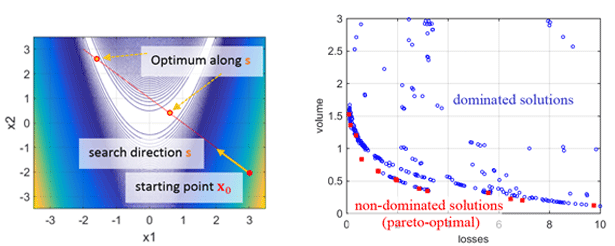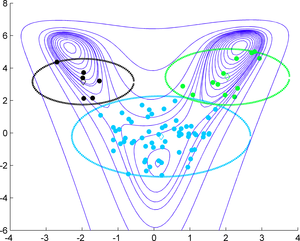# Optimization and inverse problems

Numerical optimization

This lecture mainly covers deterministic optimization approaches for finding the minimum of a scalar objective function and their mathematical background. After the introduction of higher order first and second derivatives (gradient and Hessian matrix) optimality conditions of unconstrained problems as well as problems with linear equality and inequality constraints are derived. Auxiliary methods like line search and tools for calculating gradients are discussed.

Then zero order methods (simplex and pattern search approach), second order methods (Newton method) and first order methods (conjugate gradient and quasi Newton approach) are introduced for unconstrained optimization. Data fitting problems can advantageously be treated using Gauss Newton method.

In case of linear equality constraints an elimination method based on a quadratic model, which is also an essential part of SQP (sequential quadratic programming) is derived. Besides that, the classical Lagrange method is presented. Linear inequality constraints are treated applying an active set method. Special attention is put on linear programming, where the table method is derived in detail.

Stochastic methods a briefly introduced and their principal behavior is discussed based on simple evolution strategies (ES), namely the (1+1) ES and the (µ,lambda) ES.Line search on Rosenbrock’s function (left) and pareto front of a multi-objective optimization problem (right)

Stochastic optimization methods

This lecture introduces stochastic optimization methods for the solution of multi-objective and multi-modal optimization problems. Firstly, evolutionary algorithms like evolution strategies and genetic algorithms and population based methods like particle swarm method (PSO) and fire fly method (FFO) are presented by starting with simple versions which are gradually made more complex. Setting up the objective function of multi-objective problems by weighted sums or fuzzy membership functions is tackled as well as useful representations of the behavior of (many) trial variables in the due course of the iterative optimization process.

Specially designed methods for solving multi-objective problems by treating these objectives individually to find a pareto front of non dominated solutions like NSGA (non dominated sorting genetic algorithm) are presented.

Since many components of electromagnetic devices are subject to statistical variabilities, robust optimization is of major importance and some basic approaches are discussed.

To overcome the problem of high computation times due to a high number of function calls when applying a stochastic approach, model order reduction (MOR) has become an essential feature when setting up an optimization environment. Neural networks or multi-quadrics are among the surrogate model discussed which are used to lower the computational burden.Cluster of individuals using an evolutionary algorithm